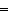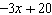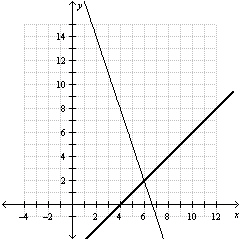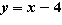Name:    Quiz B.1-B.3

Multiple Choice
Identify the choice that best completes the statement or answers the question.

Use the temperature scales to convert between Celsius and Kelvin.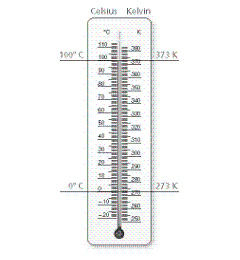1.

Kelvin is a temperature scale used by scientists. Convert 81°C  to Kelvin.
 a. 80 K c. 354 K b. 81 K d. –192 K

2.

Water boils at 100°C. At what temperature does water boil on the Kelvin scale?
 a. 373 K c. 100 K b. 99 K d. –173 K

Solve the equation. Check your solution.

3.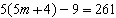a.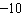c.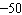b.d.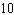4.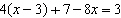a.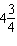c.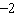b.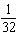d.Solve the equation. Check your solution.

5.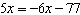a.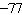c.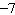b.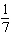d.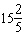6.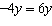a. –10y c. 0 b. 10 d. 10y

7.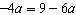a.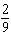c.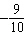b.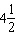d.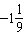8.

It costs \$120 to be a member of a music club. A member of the club pays \$5 per music lesson. A nonmember pay \$15 per music lesson. How many music lessons must a member and a nonmember take so the cost for each is the same?
 a. 6 c. 105 b. 12 d. 110

Numeric Response

1.

What is the diameter of a soup can if the lateral surface area is 703.36 square centimeters and the height is 14 centimeters?

In a regular polygon each side has the same length. Find the perimeter of the regular polygon.

2.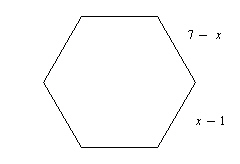Solve the equation. Check your solution.

1.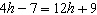Use the graph to solve the equation. Check your solution.

2.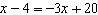y